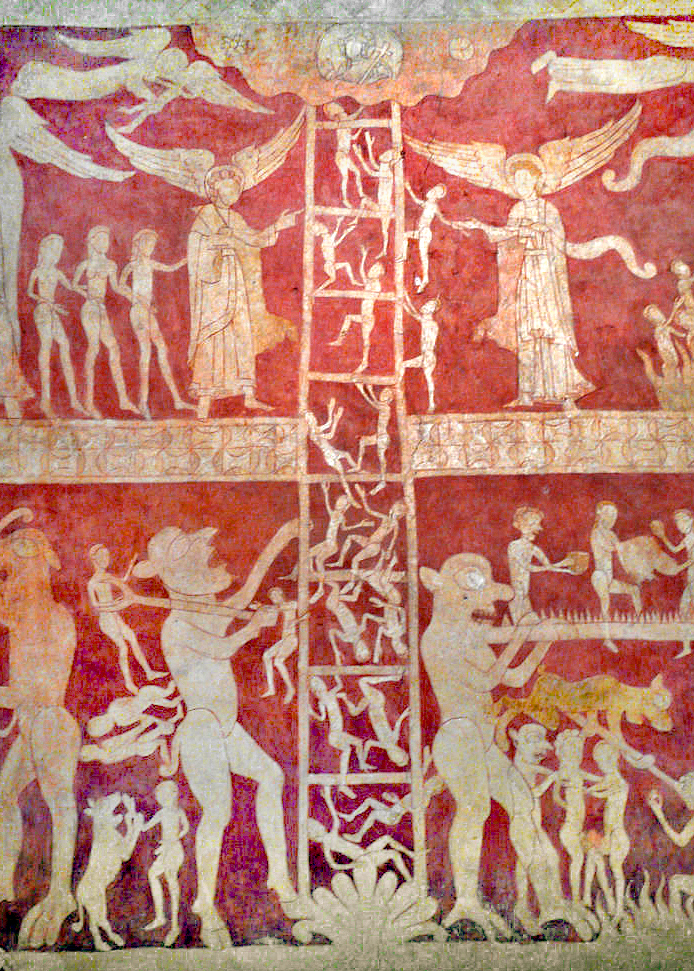# A new proof of the Barwise extension theorem, without infinitary logic, CUNY Logic Workshop, December 2018

I’ll be back in New York from Oxford, and this will be a talk for the CUNY Logic Workshop, December 14, 2018.Abstract. I shall present a new proof, with new applications, of the amazing extension theorem of Barwise (1971), which shows that every countable model of ZF has an end-extension to a model of ZFC + V=L. This theorem is both (i) a technical culmination of Barwise’s pioneering methods in admissible set theory and the admissible cover, but also (ii) one of those rare mathematical results saturated with significance for the philosophy of set theory. The new proof uses only classical methods of descriptive set theory, and makes no mention of infinitary logic. The results are directly connected with recent advances on the universal $\Sigma_1$-definable finite set, a set-theoretic version of Woodin’s universal algorithm.

## 3 thoughts on “A new proof of the Barwise extension theorem, without infinitary logic, CUNY Logic Workshop, December 2018”

1. Hi Joel,

To flesh out my comment from your Q&A session, given a countable M, consider the theory T consisting of the atomic diagram of M, together with ZFC + V = L. You proved that this theory is (finitely) consistent in your “nonstandard” case (note that in this proof, we do not need to assume that M is omega nonstandard). So by the omitting types theorem, there is a model N of T such that N is an end extension of M.

• Athar, thanks very much for this. It seems to me that this way of arguing in part amounts to replacing the Keisler-Morley theorem in my argument with the omitting types theorem. That isn’t too suprising, I suppose, since one can prove the Keisler-Morley theorem by omitting types. But your argument also unifies nicely with the rest of the argument, and so I think it is very nice. I am wondering whether this way of thinking can simply the proof of the universal $\Sigma_1$-definable sequence? The current argument is based on this proof of the Barwise extension theorem, and perhaps we can simplify it via omitting types. I want to think that through.

• Thinking a bit more about this, it isn’t clear to me yet how to prove that the types are not principal. I’ve sent you an email.